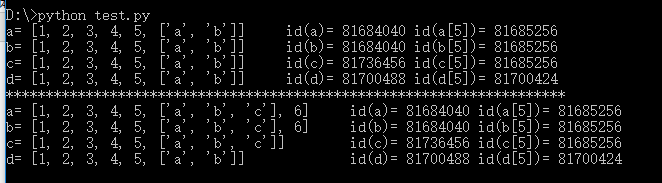# python深拷贝和浅拷贝的区别

### 首先深拷贝和浅拷贝都是对象的拷贝，都会生成一个看起来相同的对象，他们本质的区别是拷贝出来的对象的地址是否和原对象一样，也就是地址的复制还是值的复制的区别。

#encoding=utf-8

import copy
a=[1,2,3,4,5,['a','b']]
#原始对象
b=a#赋值，传对象的引用
c=copy.copy(a)#对象拷贝，浅拷贝
d=copy.deepcopy(a)#对象拷贝，深拷贝
print "a=",a,"    id(a)=",id(a),"id(a)=",id(a)
print "b=",b,"    id(b)=",id(b),"id(b)=",id(b)
print "c=",c,"    id(c)=",id(c),"id(c)=",id(c)
print "d=",d,"    id(d)=",id(d),"id(d)=",id(d)
print "*"*70

a.append(6)#修改对象a
a.append('c')#修改对象a中的['a','b']数组对象
print "a=",a,"    id(a)=",id(a),"id(a)=",id(a)
print "b=",b,"    id(b)=",id(b),"id(b)=",id(b)
print "c=",c,"       id(c)=",id(c),"id(c)=",id(c)
print "d=",d,"            id(d)=",id(d),"id(d)=",id(d)c是a进行浅拷贝生成的对象，可以看到a（或b）和c两个对象整体的id是不同的，但是里面的第5个元素-列表的地址却是相同的（指向同一个地址），所以b在浅层次元素层面（不可变）增加一个元素时，c并没跟着增加，但是b的第5个元素-列表在增加一个元素时，c的第5个元素也跟着增加了，这就是因为b和c的第5个元素-列表是指向同一个地址的，这个地址上的值变了，在两个地方会同时改变；

List1=[‘a’,’b’,’c’]

List2=[‘a’,’b’,’c’]

Id(list1)=id(list2)，但是两个列表的地址是不同的

int,float,complex,long,str,unicode,tuple

posted @ 2018-10-04 16:09  夏晓旭  阅读(45012)  评论(5编辑  收藏  举报